Code

Archaeological Site Map

Pachacuti's Golden Shield

Help
 1 2 3 4 5 6 7 8 9 10 11 12
```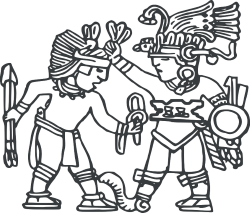key = 7
key = key + 5
key = key * 2
key = key / 3
key = key ^ 2

OUTPUT key

/* ----------------------------------
Help:
^ is the exponentiation operator:
a^b means a to the power of b.
-----------------------------------*/
```
```snake_A = 2 * (6 - 3)
snake_B = 10 - 6 / 2

IF snake_A > snake_B THEN
key = snake_A ^ 2
ELIF snake_A < snake_B THEN
key = snake_B ^ 2
ELSE
key = snake_A + snake_B
END IF

OUTPUT key
/* ----------------------------------
Help:
^ is the exponentiation operator:
a^b means a to the power of b.
-----------------------------------*/```
```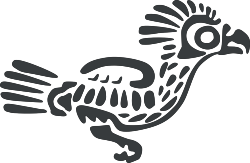key = 0
FOR i FROM 1 TO 10
key = key + 12
NEXT i

WHILE key > 20 DO
key = key / 2
END WHILE

OUTPUT key
```
```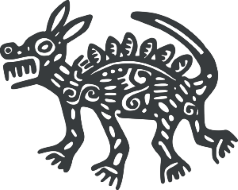primes = [2,3,5,7,11,13,17]
key = 0
count = LENGTH(primes)

FOR i FROM 0 TO count-1
key = key + primes[i]
NEXT i

OUTPUT key```
```location = "Machu Picchu"
a = LEFT(location,5)
b = RIGHT(location,6)
key= 7
IF LEFT(a,1)==LEFT(b,1) THEN
IF RIGHT(a,1)==RIGHT(b,1) THEN
key = key * 6
ELSE
key = key * 7
END IF
ELSE
IF RIGHT(a,1)==RIGHT(b,1) THEN
key = key * 8
ELSE
key = key * 9
END IF
END IF
OUTPUT key
```
```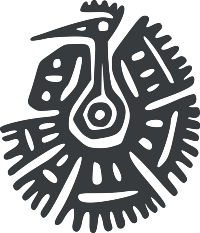FUNCTION getVolume(width,length,height)
volume = width * length * height
return volume
END FUNCTION

w = 4
l = 5
h = 7
key = getVolume(w, l, h)

OUTPUT key```
```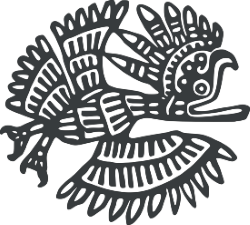key = 122

FOR i FROM 1 TO 8
FOR j FROM 1 TO 4
key = key - 1
NEXT j
NEXT i

OUTPUT Key```
```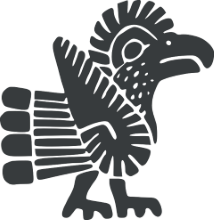a = 7
b = 2 * a
c = b - 5
if a>=b AND b<c THEN
key = a + b + c
ELSE IF a>b OR c>b THEN
key = a - b - c
ELSE IF a<=b AND b>=c
key = a + b - c
ELSE
key = a - b + c
END IF

OUTPUT key
```
```key = 0
FOR i FROM 1 TO 10
IF i MOD 2 == 0 THEN
key = key + 4
ELSE
key = key - 1
END IF
NEXT i

IF key MOD 5 == 0  THEN
key = key * 4
END IF

OUTPUT key

/* --------------------------------------
DIV is the quotient of a whole division,
MOD is the remainder of a whole division.
-----------------------------------------*/```
```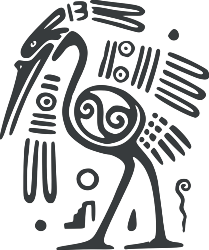FUNCTION abs(x,y)
IF x > y THEN
RETURN x - y
ELSE
RETURN y - x
END IF
END FUNCTION

a = 7 * 5
b = 24 * 2
key = abs(a,b)

OUTPUT key
```
```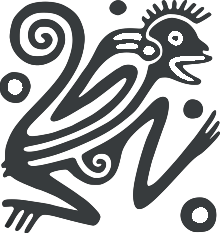code = "COCA"
key = 0

letter = LEFT(code,1)
key = key + ASCII(letter)

letter = RIGHT(code,1)
key = key + ASCII(letter)

OUTPUT Key

/* --------------------------------------
HELP:
The ASCII code for character A is 65
-----------------------------------------*/```
```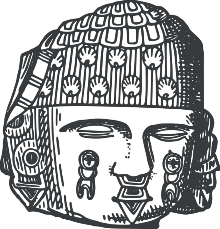FUNCTION factorial(n)
x = 1
FOR i FROM 1 TO n
x = x * i
NEXT i
RETURN x
END FUNCTION

key = factorial(5)
OUTPUT key
```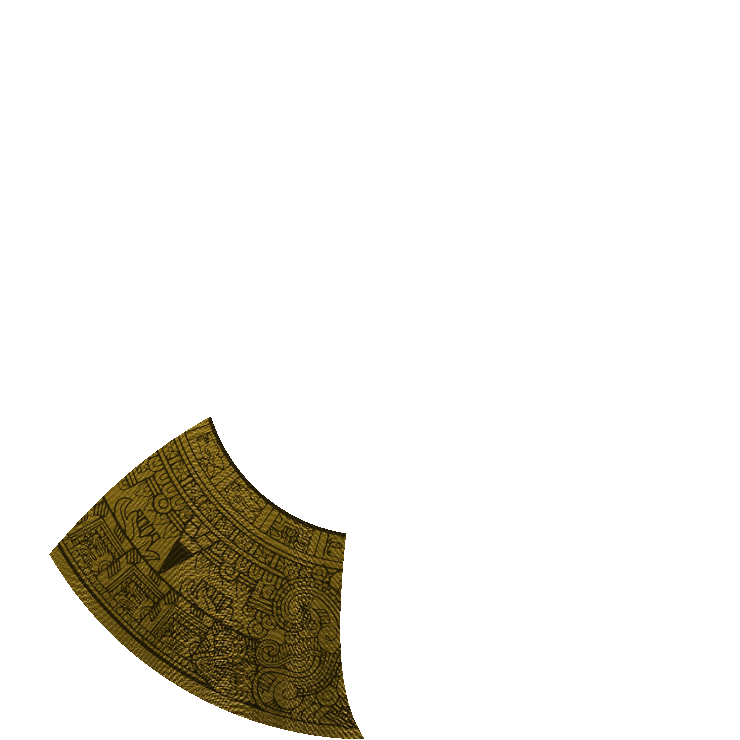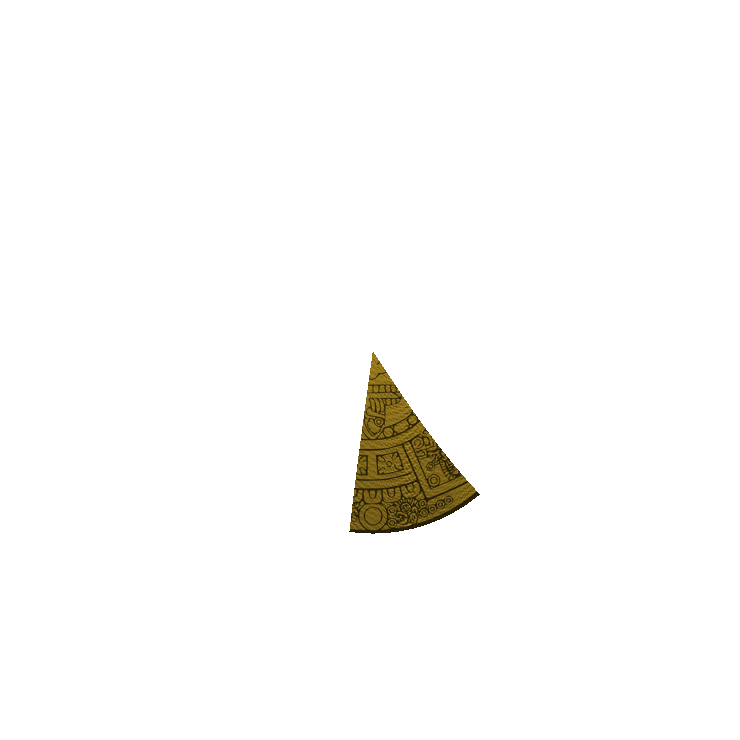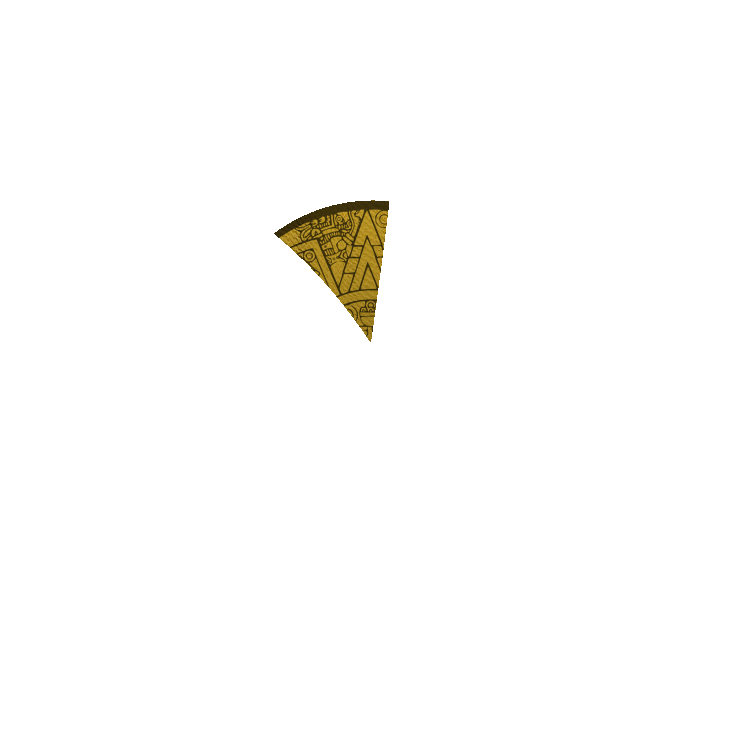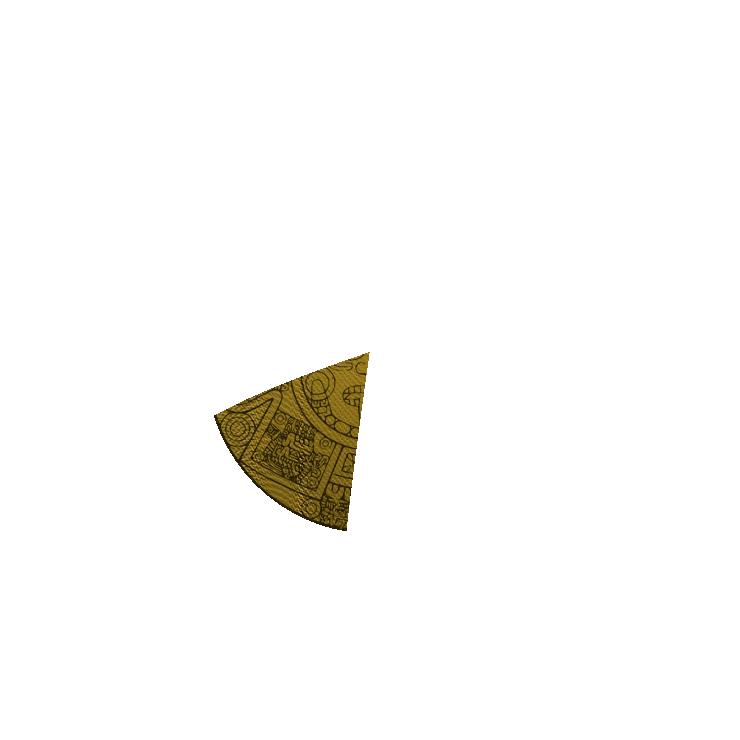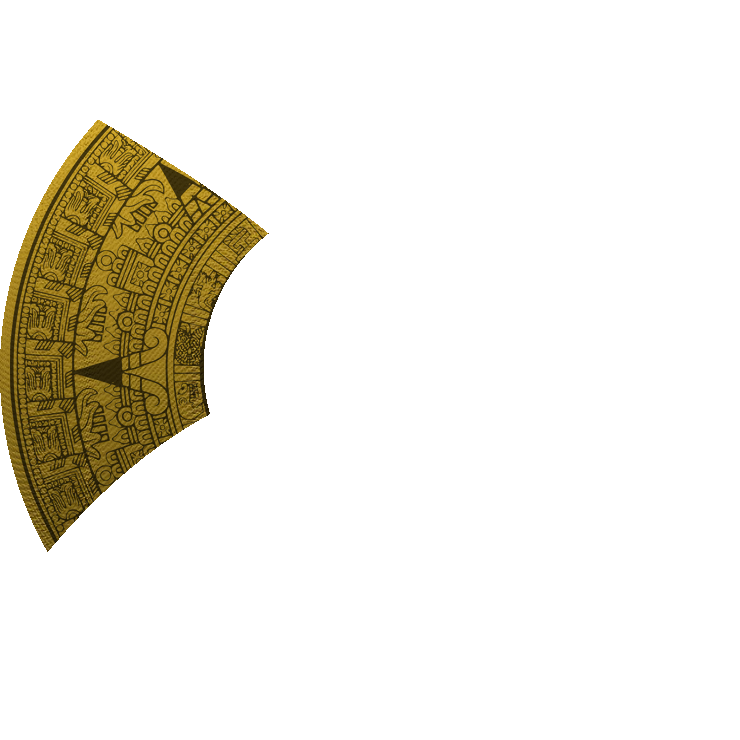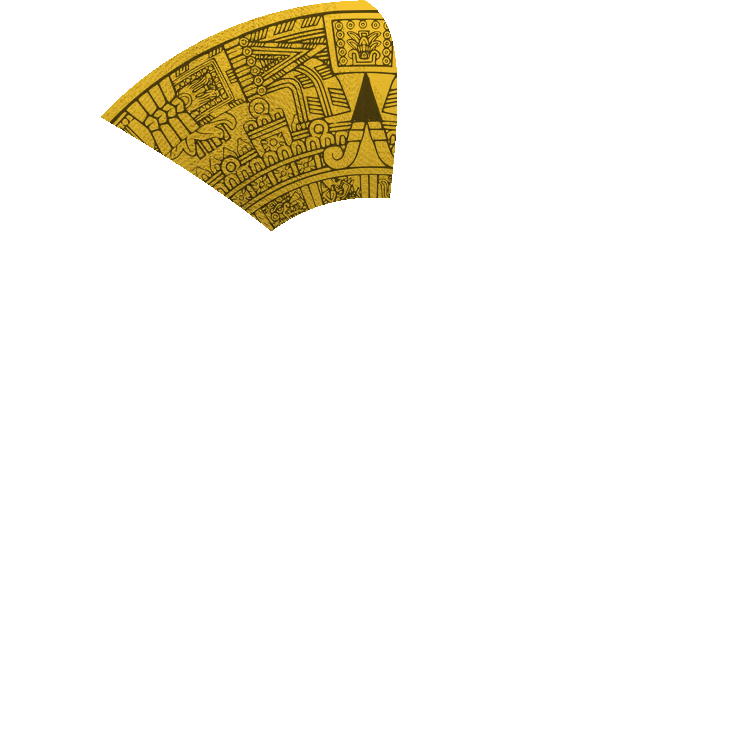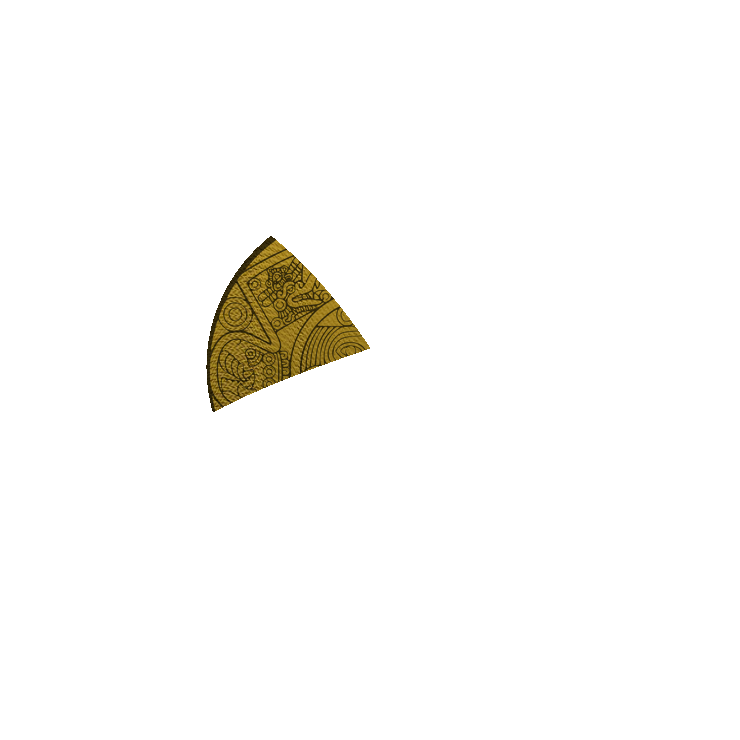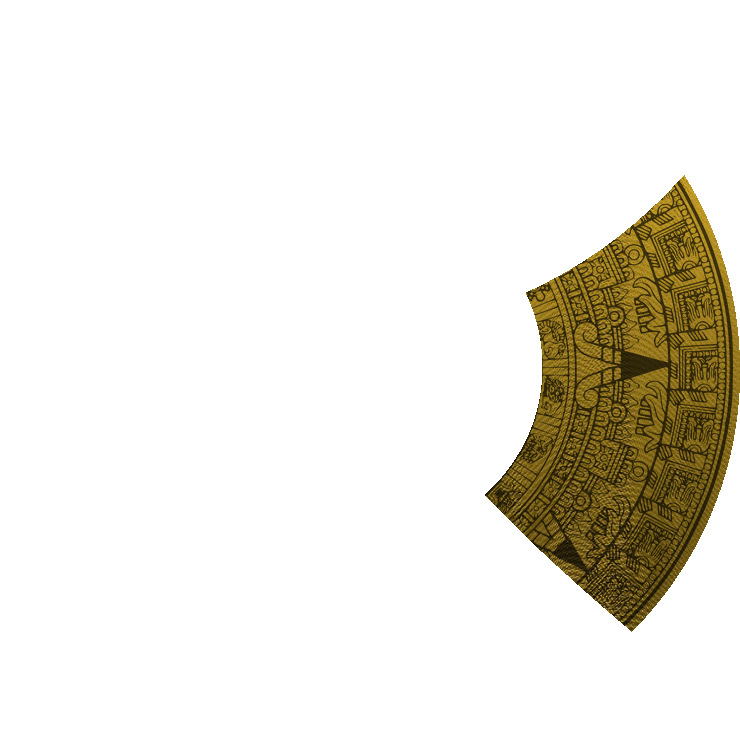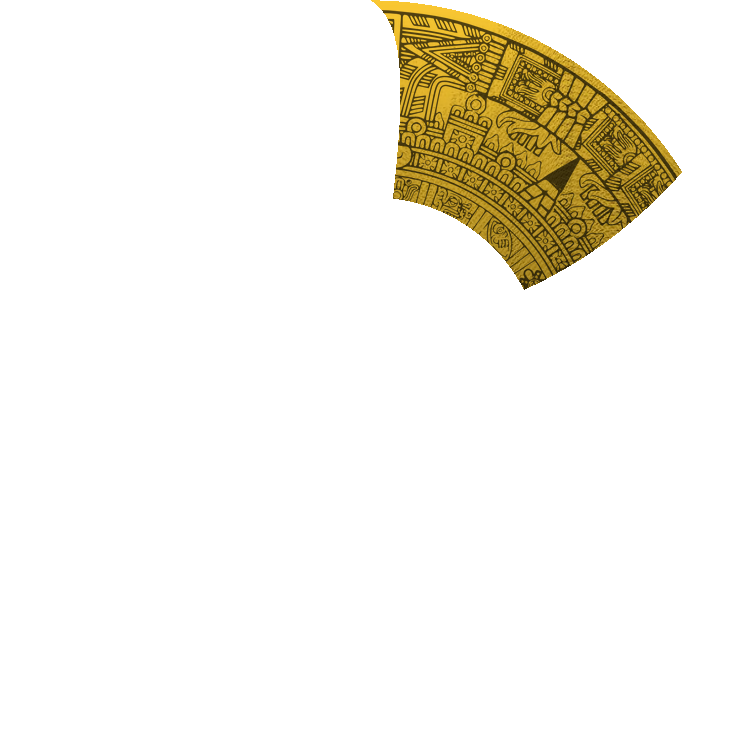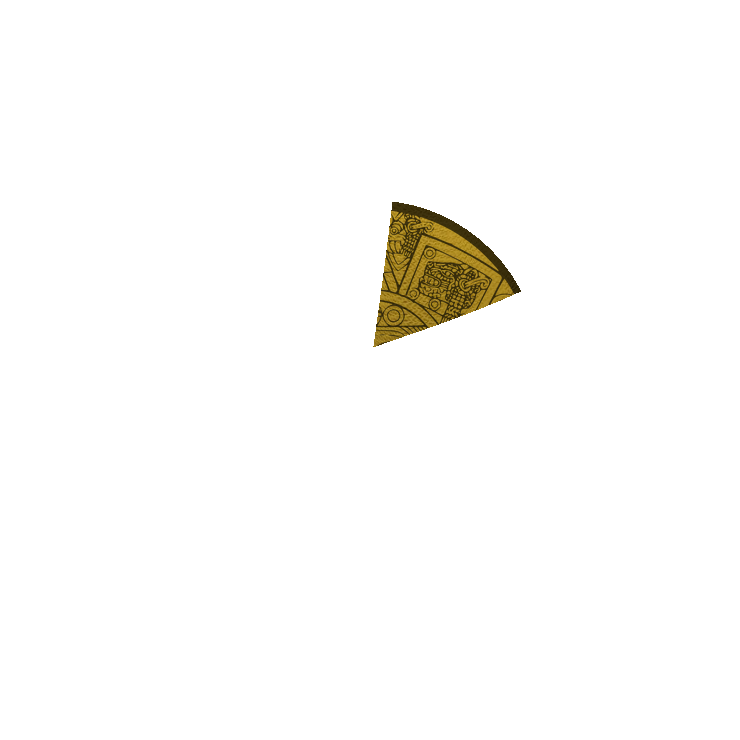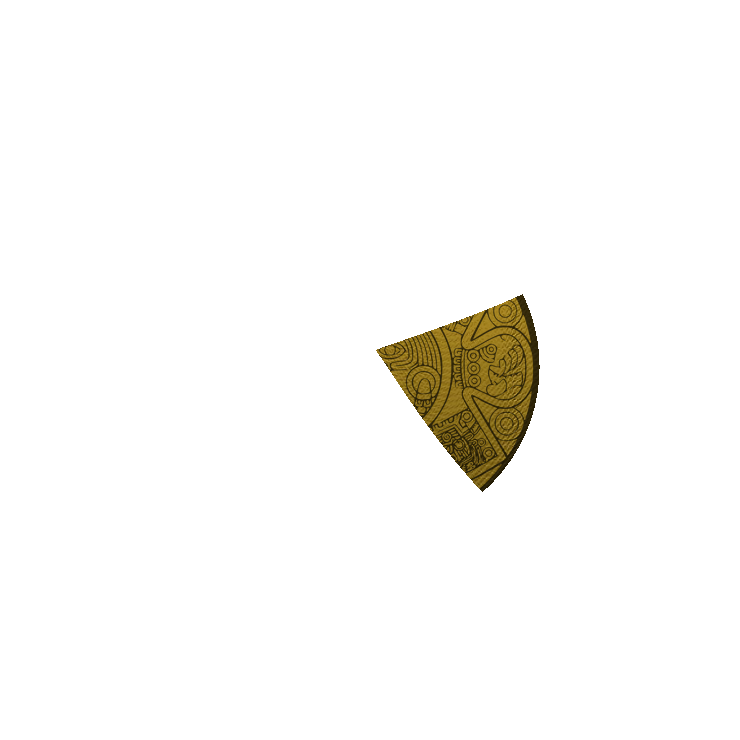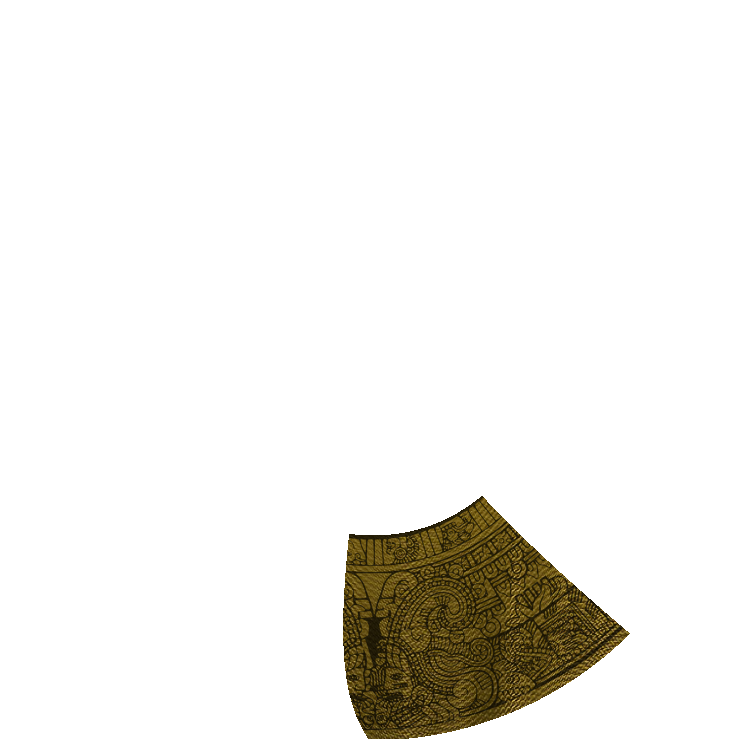There is nothing here!

You have found one piece of Pachacuti's Golden Shield:```String Manipulation
string = "abcdef"
LENGTH(string) would return the number of characters in the string (e.g. 6)
LEFT(string,3) would return the first 3 characters of the string (e.g. "abc")
RIGHT(string,3) would return the last 3 characters of the string (e.g. "def")

MOD & DIV
DIV is the quotient of a division, whereas MOD is the remainder.
For instance: 20 / 3 = 6 remainder 2
So:
20 DIV 3 would return 6
20 MOD 3 would return 2

ASCII Codes
Check the following ASCII table to lookup the right codes.
```